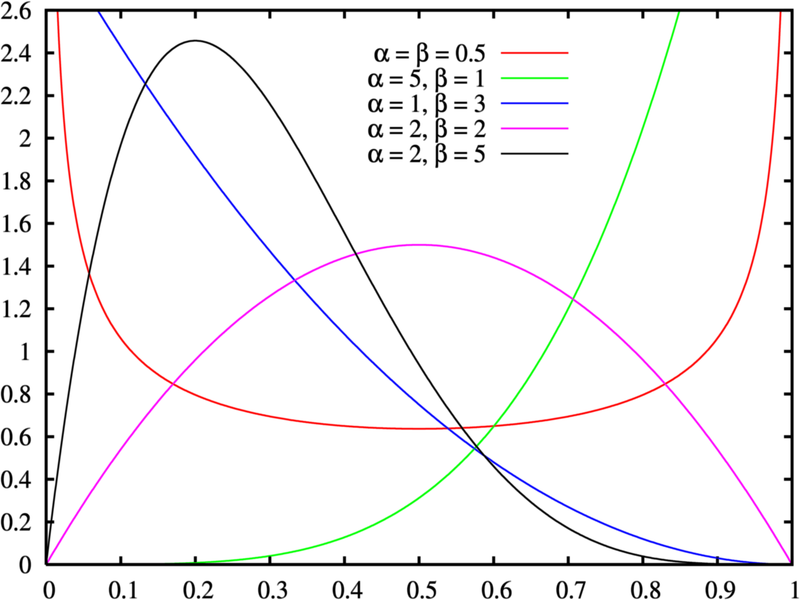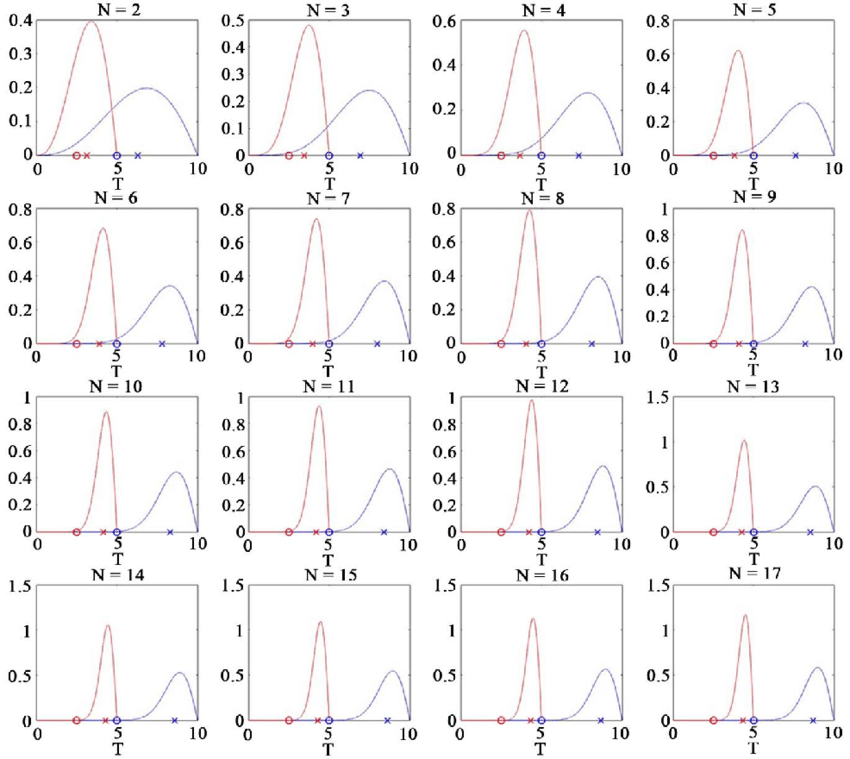# Parabolic Distribution

Share on

## What is a Parabolic Distribution?

A parabolic distribution is any distribution that has the shape of a parabola. Many different probability distributions from a wide variety of families can be parabolic in shape.

## Examples of Parabolic Distribution

In general, if a probability distribution can be modeled with a quadratic function (creating a U or upside down U), then that distribution is parabolic. For example, the following distribution , which is a quadratic function, is parabolic:
f(xi) = 6(xi – A)(B – xi) / (B – A)2.

The beta distribution can take on many different shapes, from u-shaped curves to bell curves. However, when α = β = 2, the distribution becomes parabolic.The Beta Distribution pdf, showing several different shapes with shape parameters α and β. The pink curve α = β = 2 is parabolic.

The following image shows two examples of a parabolic distribution: a = 5 (red) and a = 10 (blue). The x-axis is a random variable (T), and the y-axis shows the probability density of T .If you have a set of data that makes a parabola and you need to find the best fit equation, use quadratic regression.

Parabolic distributions of order 2 are members of the location-scale family of distributions.

## References

Graph created with Desmos.com.
 Meloun, M. & Militky, J. Errors in instrumental measurements.
 Image: Motohisa Osaka via Researchgate. CC by 4.0.

CITE THIS AS:
Stephanie Glen. "Parabolic Distribution" From StatisticsHowTo.com: Elementary Statistics for the rest of us! https://www.statisticshowto.com/parabolic-distribution/
---------------------------------------------------------------------------Need help with a homework or test question? With Chegg Study, you can get step-by-step solutions to your questions from an expert in the field. Your first 30 minutes with a Chegg tutor is free!